• 共轭转置矩阵
万次阅读 多人点赞
2018-12-04 20:08:00

实数矩阵的转置就是将行和列进行调换。实数的共轭是它本身，所以是实数矩阵的共轭转置就是它的转置矩阵，共轭矩阵不变。

复数的共轭就是将虚数取相反数，例：a+bi共轭之后就是a-bi。复数矩阵的共轭转置就是各成员取共轭然后再转置。

更多相关内容
• ## 共轭矩阵

千次阅读 2020-12-20 05:03:44
共轭方程的导出是建立资料同化模型的关键,其导出方式有两种途径:AFD形式与FDA形式.在特征线计算格式基础上针对一类较广泛海洋动力控制方程分析了其两种共轭方程(AFD形式与FDA形式)之间的关系,并将理论结果应用于波谱...

共轭方程的导出是建立资料同化模型的关键,其导出方式有两种途径:AFD形式与FDA形式.在特征线计算格式基础上针对一类较广泛海洋动力控制方程分析了其两种共轭方程(AFD形式与FDA形式)之间的关系,并将理论结果应用于波谱共轭方程的讨论.

共轭双曲线以已知双曲线的虚轴为实轴，实轴为虚轴的双曲线叫做原双曲线的共轭双曲线，通常称它们互为共轭双曲线．

共轭双曲线有共同的渐近线；

共轭双曲线的四个焦点共圆．

例 过双曲线的一个顶点的切线交共轭双曲线于两点，求证：过交点所作共轭双曲线的两切线必通过原双曲线的另一顶点．

点A′．

方程：x2/a2-y2/b2=1与y2/b2-x2/a2=1互为共轭双曲线

双曲线与椭圆有哪些不同？

(1)定义不同，图形不同。

(2)有两类特殊的双曲线，它们有一些特殊的性质。

一类是等轴双曲线。其主要性质有：a=b，离心率为根号2，两条渐近线互相垂直，等轴双曲线上任意一点到中心的距离是它到两个焦点的距离的比例中项。

另一类是共轭双曲线，其主要性质有：它们有共同的渐近线，它们的四个焦点共圆，它们的离心率的倒数的平方和等于1。

等轴双曲线是一个方程所对应的几何图形。有两支曲线：而互为共轭双曲线则是两个方程所对应的几何图形，每个方程各对应两支曲线。等轴双曲线也有它的共轭双曲线。

共轭矩阵

又称Hermite阵。Hermite阵中每一个第i 行第j 列的元素都与第j 行第i 列的元素的共轭相等。埃尔米特矩阵(或自共轭矩阵)是相对其主对角线以复共轭方式对称, 即是 ai,j=a*j,i。

对于

A

展开全文• 矩阵的迹（Trace）   n×nn\times nn×n的方阵A的n个对角线元素的和称为方阵A的迹，记作tr(A). A=(a11⋯a1n⋮ ⋮an1⋯ann)A=\begin{pmatrix}a_{11}&\cdots &a_{1n}\\\vdots&\ &\vdots\\a_{n1...

## 矩阵的迹（Trace）

n × n n\times n 的方阵A的n个对角线元素的和称为方阵A的迹，记作tr(A).

A = ( a 11 ⋯ a 1 n ⋮   ⋮ a n 1 ⋯ a n n ) A=\begin{pmatrix}a_{11}&\cdots &a_{1n}\\\vdots&\ &\vdots\\a_{n1}&\cdots&a_{nn}\end{pmatrix}
由定义知， t r ( A ) = a 11 + a 22 + ⋯ + a n n = ∑ j = 0 n a j j tr(A)=a_{11}+a_{22}+\cdots +a_{nn}=\displaystyle\sum\limits_{j=0}^n a_{jj}

## 矩阵的秩（Rank）

定义1（比较晦涩）：域 F F n × m n\times m 的非零矩阵A的所有子式中必有一个阶数最大的非零子式，其阶数称为矩阵A的秩，记作 r a n k ( A ) rank(A) . 零矩阵的秩定义为零。

定义2：矩阵 A A 的秩是 A A 的列空间的维数，记作 r a n k ( A ) rank(A) 。（因为 A A 的主元列形成 A A 的列空间 C o l   A Col\space A 的一个基，所以 A A 的秩正好是 A A 的主元列的个数。）

由于矩阵A的子式阶数不超过A的行数及列数，所以域 F F n × m n\times m 的非零矩阵A的秩有：

（1） 0 ≤ r a n k ( A ) ≤ m i n ( n , m ) 0\leq rank(A)\leq min(n,m) ;
（2） r a n k ( c A ) = r a n k ( A ) rank(cA)=rank(A) ，其中c为不等于0的常数；
（3） r a n k ( A T ) = r a n k ( A ) rank(A^T)=rank(A) ;
（4） r a n k ( A ‾ ) = r a n k ( A ) rank(\overline{A})=rank(A) , r a n k ( A ∗ ) = r a n k ( A ) rank(A^{*})=rank(A) . 其中 A ‾ \overline{A} A A 的共轭矩阵， A ∗ A^{*} A A 的伴随矩阵。

#### 使用MATLAB求矩阵的秩(RANK)

若要求矩阵 A A 的秩，可以先将矩阵 A A 化简为简化阶梯阵（reduced echelon form），然后数其主元列（pivot columns）数即为 A A 的秩。

>>A = [1 2 3 4;4 5 6 7;6 9 12 15;1 1 1 1]
A =
1              2              3              4
4              5              6              7
6              9             12             15
1              1              1              1

>>rref(A)   //求简化阶梯阵
ans =
1              0             -1             -2
0              1              2              3
0              0              0              0
0              0              0              0
//可见A的简化阶梯阵有2个主元列---第一列和第二列，所以秩为2. 可以使用rank()函数快速求矩阵的秩，进行验算

>>rank(A)
ans =
2
//验算结果和计算的主元列数一致。


可见，对于上例的矩阵 A A ，有两个主元列（第一和第二列），所以秩为2。由于上述矩阵化为简化阶梯阵后，有2列不是主元列，所以有方程 A x = 0 Ax=0 有2个自由变量（非主元列对应于方程 A x = 0 Ax=0 的自由变量），所以主元列加非主元列正好等于矩阵 A A 的列数。所以可以得出一下秩定理：

### 共轭矩阵（Conjugate Matrix）

n × m n\times m 的复矩阵A的共轭矩阵是 m × n m\times n 的矩阵（形如其转置矩阵），记作 A ∗ A^{*}
A = ( a 11 ⋯ a 1 m ⋮   ⋮ a n 1 ⋯ a n m ) A=\begin{pmatrix}a_{11}&\cdots &a_{1m}\\\vdots&\ &\vdots\\a_{n1}&\cdots&a_{nm}\end{pmatrix}
A ‾ = ( a 11 ‾ ⋯ a 1 m ‾ ⋮   ⋮ a n 1 ‾ ⋯ a n m ‾ ) \overline{A}=\begin{pmatrix}\overline{a_{11}}&\cdots &\overline{a_{1m}}\\\vdots&\ &\vdots\\\overline{a_{n1}}&\cdots&\overline{a_{nm}}\end{pmatrix}
A ∗ = ( a 11 ‾ ⋯ a n 1 ‾ ⋮   ⋮ a 1 m ‾ ⋯ a n m ‾ ) A^{*}=\begin{pmatrix}\overline{a_{11}}&\cdots &\overline{a_{n1}}\\\vdots&\ &\vdots\\\overline{a_{1m}}&\cdots&\overline{a_{nm}}\end{pmatrix}

共轭矩阵求法是，先将A转置，再对每个元素求共轭即可：
A ∗ = A T ‾ A^{*}=\overline{A^T}

共轭矩阵也叫自共轭矩阵、Hermite矩阵，要求元素 a j k a_{jk} a k j a_{kj} 共轭，即 a j k ‾ = a k j ‾ \overline{a_{jk}}=\overline{a_{kj}} 如：
( 1 3 + 2 i 3 − 2 i 1 ) \begin{pmatrix}1&3+2i\\3-2i&1\end{pmatrix} 中， a 12 = 3 + 2 i a_{12}=3+2i a 21 = 3 − 2 i a_{21}=3-2i 共轭相等，所以此矩阵为共轭矩阵。

设矩阵A为域F上的n阶方阵：
A = ( a 11 ⋯ a 1 n ⋮   ⋮ a n 1 ⋯ a n n ) A=\begin{pmatrix}a_{11}&\cdots &a_{1n}\\\vdots&\ &\vdots\\a_{n1}&\cdots&a_{nn}\end{pmatrix}
A j k A_{jk} 为元素 a j k a_{jk} 的代数余子式，用域F上 n 2 n^2 个数 A j k A_{jk} 构成的n阶方阵即方阵A的伴随方阵，记作 A ( ∗ ) A^{(*)} 。求法，先构成代数余子式矩阵：
( A 11 A 12 ⋯ A 1 n A 21 A 22 ⋮ A 2 n ⋮ ⋮   ⋮ A n 1 A n 2 ⋯ A n n ) \begin{pmatrix}A_{11}&A_{12}&\cdots &A_{1n}\\A_{21}&A_{22}&\vdots&A_{2n}\\\vdots&\vdots&\ &\vdots\\A_{n1}&A_{n2}&\cdots&A_{nn}\end{pmatrix}

再将其转置即可得到A的伴随矩阵 A ( ∗ ) A^{(*)}

( A 11 A 21 ⋯ A n 1 A 12 A 22 ⋮ A n 2 ⋮ ⋮   ⋮ A 1 n A 2 n ⋯ A n n ) \begin{pmatrix}A_{11}&A_{21}&\cdots &A_{n1}\\A_{12}&A_{22}&\vdots&A_{n2}\\\vdots&\vdots&\ &\vdots\\A_{1n}&A_{2n}&\cdots&A_{nn}\end{pmatrix}

## 基底（Basis）维数（Dimension）与秩（Rank）

基底（或称基，basis）：如果向量空间 H H 中的一组线性无关向量可以把该空间内所有向量通过线性组合的方式表示出来，那么这组向量成为该空间的一个基。
由定义知，基是一组向量的组合。

相对某个基的坐标的计算方法：如果 B = { b 1 , … , b p } B=\{b_1,\dots ,b_p\} 是子空间 H H 的一组基，对 H H 中每一个向量 x x ，相对于基 B = { b 1 , … , b p } B=\{b_1,\dots ,b_p\} 的坐标是使得 x = c 1 b 1 + ⋯ + c p b p x=c_1b_1+\dots +c_pb_p 成立的权 c 1 , … , c p c_1,\dots,c_p ，且 R p R^p 中的向量 [ x ] B = [ c 1 ⋮ c p ] [x]_B=\begin{bmatrix}c_1\\\vdots\\c_p\end{bmatrix} 称为 x x 相对于基 B B 的坐标向量，或称 x x B B -坐标向量。

：设 v 1 = [ 3 6 2 ] v_1=\begin{bmatrix}3\\6\\2\end{bmatrix} v 2 = [ − 1 0 1 ] v_2=\begin{bmatrix}-1\\0\\1\end{bmatrix} x = [ 3 12 7 ] x=\begin{bmatrix}3\\12\\7\end{bmatrix} B = { v 1 , v 2 } B=\{v_1,v_2\} ，因为 v 1 , v 2 v_1,v_2 显然线性无关，所以 B B H = S p a n { v 1 , v 2 } H=Span\{v_1,v_2\} 的基。请判断 x x 是否在空间 H H 中，如果在，请求出 x x 相对于 B B 的坐标向量。

解：

如果 x x 在空间 H H 中（空间 H H 是v_1和v_2确定的平面），则方程 c 1 v 1 + c 2 v 2 = x c_1v_1+c_2v_2=x c 1 c_1 c 2 c_2 称为权，即：

c 1 [ 3 6 2 ] + c 2 [ − 1 0 1 ] = [ 3 12 7 ] c_1\begin{bmatrix}3\\6\\2\end{bmatrix}+c_2\begin{bmatrix}-1\\0\\1\end{bmatrix}=\begin{bmatrix}3\\12\\7\end{bmatrix} 是有解的（或说相容的）

如果权 c 1 c_1 c 2 c_2 存在，那么它们是 x x B B -坐标。

下面解方程： c 1 v 1 + c 2 v 2 = x c_1v_1+c_2v_2=x 对应的增广矩阵：

[ 3 − 1 3 6 0 12 2 1 7 ] → [ 1 0 2 0 1 3 0 0 0 ] \begin{bmatrix}3&-1 &3\\6&0&12\\2&1&7\end{bmatrix}\rightarrow \begin{bmatrix}1&0&2\\0&1&3\\0&0&0\end{bmatrix}

即： c = [ c 1 c 2 ] = [ 2 3 ] c=\begin{bmatrix}c_1\\c_2\end{bmatrix}=\begin{bmatrix}2\\3\end{bmatrix}

所以 [ x ] B = [ c 1 c 2 ] = [ 2 3 ] [x]_B=\begin{bmatrix}c_1\\c_2\end{bmatrix}=\begin{bmatrix}2\\3\end{bmatrix} 就是 x x 相对于基 B B 的坐标向量，或称 x x B B -坐标向量。

即： x = 2 v 1 + 3 v 2 x=2v_1+3v_2 ，画图如下：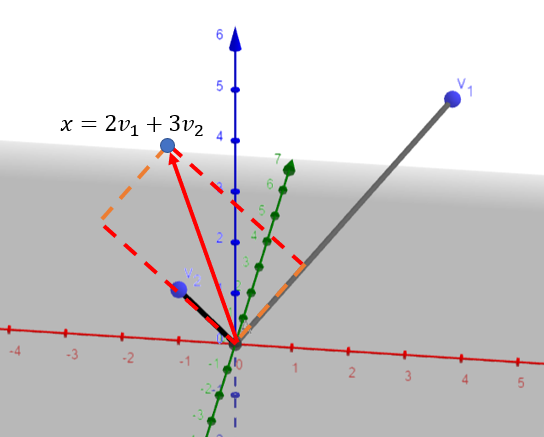总结：

虽然 H H 中的点也在 R 3 R^3 中，但是它们完全由属于 R 2 R^2 的坐标向量确定（因为本例中 H H 是v_1和v_2确定的平面，属于 R 2 R^2 ）。映射 x ∣ → [ x ] B x|\rightarrow [x]_B 是使 H H R 2 R^2 之间保持线性组合关系的一一映射，那么可以称这种映射是同构的，本例中 H H R 2 R^2 同构。

一般地，如果 B = { b 1 , … , b p } B=\{b_1,\dots ,b_p\} 是子空间 H H 的一组基，则映射 x ∣ → [ x ] B x|\rightarrow [x]_B 是使 H H R p R^p 的形态一样的一一映射（ H H 中的向量可能多于 p p 个元素）。

展开全文matlab
• 旋转矩阵1. 旋转矩阵简介 1. 旋转矩阵简介 旋转矩阵维基百科 在线性代数中，旋转矩阵是用于在欧几里得空间中进行旋转的矩阵。例如，使用下面的约定， 矩阵：R=[cos(θ)−sin(θ)sin(θ)cos(θ)]R=\begin{bmatrix}...

# 1.旋转矩阵

## 1. 旋转矩阵简介

• 旋转矩阵（英语：Rotation matrix）是在乘以一个向量的时候有改变向量的方向但不改变大小的效果并保持了手性的矩阵。旋转矩阵不包括点反演，点反演可以改变手性，也就是把右手坐标系改变成左手坐标系或反之。所有旋转加上反演形成了正交矩阵 ( Q T = Q − 1 ⇔ Q T Q = Q Q T = I .   ⁣ Q^{T}=Q^{-1}\Leftrightarrow Q^{T}Q=QQ^{T}=I.\,\! )的集合。旋转可分为主动旋转与被动旋转。主动旋转是指将向量逆时针围绕旋转轴所做出的旋转。被动旋转是对坐标轴本身进行的逆时针旋转，它相当于主动旋转的逆操作。

## 2. 性质

M {\displaystyle \mathbf {M} } 是任何维的一般旋转矩阵: M ∈ R n × n {\displaystyle \mathbf {M} \in \mathbb {R} ^{n\times n}}

• 两个向量的点积(内积)在它们都被一个旋转矩阵操作之后保持不变:
a ⊤ ⋅ b = ( M a ) ⊤ ⋅ M b {\displaystyle \mathbf {a} ^{\top }\cdot \mathbf {b} =\mathbf {(Ma)} ^{\top }\cdot \mathbf {M} \mathbf {b} }
• 从而得出旋转矩阵的逆矩阵是它的转置矩阵:
M   M − 1 = M   M ⊤ = I \mathbf {M} \,\mathbf {M} ^{-1}=\mathbf {M} \,\mathbf {M} ^{\top }={\mathcal {I}} ，这里的 I {\displaystyle {\mathcal {I}}} 是单位矩阵。
• 一个矩阵是旋转矩阵，当且仅当它是正交矩阵并且它的行列式是单位一。正交矩阵的行列式是 ±1；如果行列式是 −1，则它包含了一个反射而不是真旋转矩阵。
• 旋转矩阵是正交矩阵，如果它的列向量形成 R n {\displaystyle \mathbb {R} ^{n}} 的一个正交基，就是说在任何两个列向量之间的标量积是零(正交性)而每个列向量的大小是单位一(单位向量)。
• 任何旋转向量可以表示为斜对称矩阵( A T = − A A^T = − A ) A的指数: M = exp ⁡ ( A ) = ∑ k = 0 ∞ A k k ! \mathbf {M} =\exp(\mathbf {A} )=\sum _{k=0}^{\infty }{\frac {\mathbf {A} ^{k}}{k!}}
这里的指数是以泰勒级数定义的而 A k {\displaystyle \mathbf {A} ^{k}} 是以矩阵乘法定义的。A 矩阵叫做旋转的“生成元”。旋转矩阵的李代数是它的生成元的代数，它就是斜对称矩阵的代数。生成元可以通过 M 的矩阵对数来找到。

## 3. 二维空间

• 在二维中，标准旋转矩阵具有以下形式： R ( θ ) = [ cos ⁡ θ − sin ⁡ θ sin ⁡ θ cos ⁡ θ ] {\displaystyle R(\theta )={\begin{bmatrix}\cos \theta &-\sin \theta \\\sin \theta &\cos \theta \\\end{bmatrix}}}
• 通过以下矩阵乘法旋转列向量： [ x ′ y ′ ] = [ cos ⁡ θ − sin ⁡ θ sin ⁡ θ cos ⁡ θ ] [ x y ] {{\displaystyle {\begin{bmatrix}x'\\y'\\\end{bmatrix}}={\begin{bmatrix}\cos \theta &-\sin \theta \\\sin \theta &\cos \theta \\\end{bmatrix}}{\begin{bmatrix}x\\y\\\end{bmatrix}}}}
• 一般而言，旋转后的点（x，y）的新坐标（x’，y’）为： x ′ = x cos ⁡ θ − y sin ⁡ θ   y ′ = x sin ⁡ θ + y cos ⁡ θ   {{\displaystyle {\begin{aligned}x'&=x\cos \theta -y\sin \theta \,\\y'&=x\sin \theta +y\cos \theta \,\end{aligned}}}}
• 如果θ为正（例如90°），矢量旋转方向为逆时针；如果θ为负（例如-90°），矢量旋转方向为顺时针。因此，顺时针旋转矩阵为: R ( − θ ) = [ cos ⁡ θ sin ⁡ θ − sin ⁡ θ cos ⁡ θ ]   {{\displaystyle R(-\theta )={\begin{bmatrix}\cos \theta &\sin \theta \\-\sin \theta &\cos \theta \\\end{bmatrix}}\,}}

### 3.1 普通旋转

• 矩阵特别旋转90°，180°，270°： [ − 1 0 0 − 1 ] , [ 0 1 − 1 0 ] [ 0 1 − 1 0 ] { {\displaystyle {\begin{bmatrix}-1&0\\[3pt]0&-1\\\end{bmatrix}}}, {\displaystyle {\begin{bmatrix}0&1\\[3pt]-1&0\\\end{bmatrix}}} {\displaystyle {\begin{bmatrix}0&1\\[3pt]-1&0\\\end{bmatrix}}}}

### 3.2 复平面

• 由于： ( 0 1 − 1 0 ) 2   =   ( − 1 0 0 − 1 ) { {\displaystyle {\begin{pmatrix}0&1\\-1&0\end{pmatrix}}^{2}\ =\ {\begin{pmatrix}-1&0\\0&-1\end{pmatrix}}}} ，矩阵平面 ( x y − y x ) {\displaystyle {\begin{pmatrix}x&y\\-y&x\end{pmatrix}}} 是复数平面的同类型。
•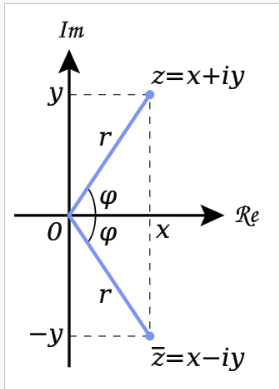z和其共轭z̅在复平面上的几何表示。从原点到点z沿淡蓝色线的距离是z的模数或绝对值。角度φ是z的自变量。

## 4. 三维空间

• 在三维空间中，旋转矩阵有一个等于单位1的实特征值。旋转矩阵指定关于对应的特征向量的旋转(欧拉旋转定理)。如果旋转角是 θ，则旋转矩阵的另外两个(复数)特征值是 exp(iθ) 和 exp(-iθ)。从而得出 3 维旋转的迹数等于 1 + 2 cos(θ)，这可用来快速的计算任何 3 维旋转的旋转角。
• 3 维旋转矩阵的生成元是三维斜对称矩阵。因为只需要三个实数来指定 3 维斜对称矩阵，得出只用三个实数就可以指定一个 3 维旋转矩阵。

### 4.1 旋转

生成旋转矩阵的一种简单方式是把它作为三个基本旋转的序列复合。关于右手笛卡尔坐标系的 x-, y- 和 z-轴的旋转分别叫做 roll, pitch 和 yaw 旋转。因为这些旋转被表达为关于一个轴的旋转，它们的生成元很容易表达。

• 绕 x-轴的主动旋转定义为:
R x ( θ x ) = [ 1 0 0 0 cos ⁡ θ x − sin ⁡ θ x 0 sin ⁡ θ x cos ⁡ θ x ] = exp ⁡ ( θ x [ 0 0 0 0 0 − 1 0 1 0 ] ) {\displaystyle {\mathcal {R}}_{x}(\theta _{x})={\begin{bmatrix}1&0&0\\0&\cos {\theta _{x}}&-\sin {\theta _{x}}\\0&\sin {\theta _{x}}&\cos {\theta _{x}}\end{bmatrix}}=\exp \left(\theta _{x}{\begin{bmatrix}0&0&0\\0&0&-1\\0&1&0\end{bmatrix}}\right)} ，这里的 θ x {\displaystyle \theta _{x}} 是 roll 角，和右手螺旋的方向相同（在yz平面逆时针）
• 绕 y-轴的主动旋转定义为:
R y ( θ y ) = [ cos ⁡ θ y 0 sin ⁡ θ y 0 1 0 − sin ⁡ θ y 0 cos ⁡ θ y ] = exp ⁡ ( θ y [ 0 0 1 0 0 0 − 1 0 0 ] ) {\displaystyle {\mathcal {R}}_{y}(\theta _{y})={\begin{bmatrix}\cos {\theta _{y}}&0&\sin {\theta _{y}}\\0&1&0\\-\sin {\theta _{y}}&0&\cos {\theta _{y}}\end{bmatrix}}=\exp \left(\theta _{y}{\begin{bmatrix}0&0&1\\0&0&0\\-1&0&0\end{bmatrix}}\right)} ，这里的 θ y {\displaystyle \theta _{y}} 是 pitch 角，和右手螺旋的方向相同（在zx平面逆时针）
• 绕 z-轴的主动旋转定义为:
R z ( θ z ) = [ cos ⁡ θ z − sin ⁡ θ z 0 sin ⁡ θ z cos ⁡ θ z 0 0 0 1 ] = exp ⁡ ( θ z [ 0 − 1 0 1 0 0 0 0 0 ] ) {\displaystyle {\mathcal {R}}_{z}(\theta _{z})={\begin{bmatrix}\cos {\theta _{z}}&-\sin {\theta _{z}}&0\\\sin {\theta _{z}}&\cos {\theta _{z}}&0\\0&0&1\end{bmatrix}}=\exp \left(\theta _{z}{\begin{bmatrix}0&-1&0\\1&0&0\\0&0&0\end{bmatrix}}\right)} ，这里的 θ z {\displaystyle \theta _{z}} 是 yaw 角，和右手螺旋的方向相同（在xy平面逆时针）。

在飞行动力学中，roll, pitch 和 yaw 角通常分别采用符号 γ , α , β \gamma,\alpha,\beta ；但是为了避免混淆于欧拉角这里使用符号 θ x , θ y 和 θ z {\displaystyle \theta _{x}} , {\displaystyle \theta _{y}} 和 {\displaystyle \theta _{z}}

### 4.2 角-轴表示和四元数表示

在三维中，旋转可以通过单一的旋转角 θ \theta 和所围绕的单位向量方向 v ^ = ( x , y , z ) {\displaystyle {\hat {\mathbf {v} }}=(x,y,z)} 来定义。
M ( v ^ , θ ) = [ cos ⁡ θ + ( 1 − cos ⁡ θ ) x 2 ( 1 − cos ⁡ θ ) x y − ( sin ⁡ θ ) z ( 1 − cos ⁡ θ ) x z + ( sin ⁡ θ ) y ( 1 − cos ⁡ θ ) y x + ( sin ⁡ θ ) z cos ⁡ θ + ( 1 − cos ⁡ θ ) y 2 ( 1 − cos ⁡ θ ) y z − ( sin ⁡ θ ) x ( 1 − cos ⁡ θ ) z x − ( sin ⁡ θ ) y ( 1 − cos ⁡ θ ) z y + ( sin ⁡ θ ) x cos ⁡ θ + ( 1 − cos ⁡ θ ) z 2 ] {\mathcal {M}}({\hat {\mathbf {v} }},\theta )={\begin{bmatrix}\cos \theta +(1-\cos \theta )x^{2}&(1-\cos \theta )xy-(\sin \theta )z&(1-\cos \theta )xz+(\sin \theta )y\1-\cos \theta )yx+(\sin \theta )z&\cos \theta +(1-\cos \theta )y^{2}&(1-\cos \theta )yz-(\sin \theta )x\\(1-\cos \theta )zx-(\sin \theta )y&(1-\cos \theta )zy+(\sin \theta )x&\cos \theta +(1-\cos \theta )z^{2}\end{bmatrix}} ### 4.3 欧拉角表示 在三维空间中，旋转可以通过三个欧拉角 ( α , β , γ ) (\alpha ,\beta ,\gamma )} 来定义。有一些可能的欧拉角定义，每个都可以依据 roll, pitch 和 yaw 的复合来表达。依据 “x-y-z” 欧拉角，在右手笛卡尔坐标中的旋转矩阵可表达为: M ( α , β , γ ) = [ cos ⁡ γ − sin ⁡ γ 0 sin ⁡ γ cos ⁡ γ 0 0 0 1 ] [ cos ⁡ β 0 sin ⁡ β 0 1 0 − sin ⁡ β 0 cos ⁡ β ] [ 1 0 0 0 cos ⁡ α − sin ⁡ α 0 sin ⁡ α cos ⁡ α ] = [ cos ⁡ γ cos ⁡ β − sin ⁡ γ cos ⁡ γ sin ⁡ β sin ⁡ γ cos ⁡ β cos ⁡ γ sin ⁡ γ sin ⁡ β − sin ⁡ β 0 cos ⁡ β ] [ 1 0 0 0 cos ⁡ α − sin ⁡ α 0 sin ⁡ α cos ⁡ α ] = [ cos ⁡ γ cos ⁡ β − sin ⁡ γ cos ⁡ α + cos ⁡ γ sin ⁡ β sin ⁡ α sin ⁡ γ sin ⁡ α + cos ⁡ γ sin ⁡ β cos ⁡ α sin ⁡ γ cos ⁡ β cos ⁡ γ cos ⁡ α + sin ⁡ γ sin ⁡ β sin ⁡ α − cos ⁡ γ sin ⁡ α + sin ⁡ γ sin ⁡ β cos ⁡ α − sin ⁡ β cos ⁡ β sin ⁡ α cos ⁡ β cos ⁡ α ] {\begin{aligned}{\mathcal {M}}(\alpha ,\beta ,\gamma )&={\begin{bmatrix}\cos \gamma &-\sin \gamma &0\\\sin \gamma &\cos \gamma &0\\0&0&1\end{bmatrix}}{\begin{bmatrix}\cos \beta &0&\sin \beta \\0&1&0\\-\sin \beta &0&\cos \beta \end{bmatrix}}{\begin{bmatrix}1&0&0\\0&\cos \alpha &-\sin \alpha \\0&\sin \alpha &\cos \alpha \end{bmatrix}}\\&={\begin{bmatrix}\cos \gamma \cos \beta &-\sin \gamma &\cos \gamma \sin \beta \\\sin \gamma \cos \beta &\cos \gamma &\sin \gamma \sin \beta \\-\sin \beta &0&\cos \beta \end{bmatrix}}{\begin{bmatrix}1&0&0\\0&\cos \alpha &-\sin \alpha \\0&\sin \alpha &\cos \alpha \end{bmatrix}}\\&={\begin{bmatrix}\cos \gamma \cos \beta &-\sin \gamma \cos \alpha +\cos \gamma \sin \beta \sin \alpha &\sin \gamma \sin \alpha +\cos \gamma \sin \beta \cos \alpha \\\sin \gamma \cos \beta &\cos \gamma \cos \alpha +\sin \gamma \sin \beta \sin \alpha &-\cos \gamma \sin \alpha +\sin \gamma \sin \beta \cos \alpha \\-\sin \beta &\cos \beta \sin \alpha &\cos \beta \cos \alpha \end{bmatrix}}\end{aligned}}} ### 4.4 对称保持 SVD 表示 对旋转轴 q q} 和旋转角 θ \theta } ，旋转矩阵 M = q q T + Q G Q T {\mathcal {M}}=qq^{T}+QGQ^{T}} ## 5 .其他 ### 5.1 旋转轴 如果使用标准的右手笛卡尔坐标系，则x轴朝右，y轴朝上，则旋转R（θ）为逆时针方向。 如果使用左手笛卡尔坐标系，其中x指向右侧但y指向下方，则R（θ）为顺时针方向。 这样的非标准方向在数学中很少使用，但在2D计算机图形学中很常见，这些图形的原点通常位于屏幕或页面的左上角和y轴上。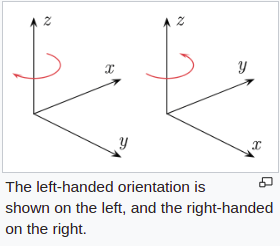### 5.2 叉乘计算 ∥ a × b ∥ = ∥ a ∥ ∥ b ∥ sin ⁡ θ . \left\|\mathbf {a} \times \mathbf {b} \right\|=\left\|\mathbf {a} \right\|\left\|\mathbf {b} \right\|\sin \theta .} d d t ( a × b ) = d a d t × b + a × d b d t , {\frac {d}{dt}}(\mathbf {a} \times \mathbf {b} )={\frac {d\mathbf {a} }{dt}}\times \mathbf {b} +\mathbf {a} \times {\frac {d\mathbf {b} }{dt}},} a × b = [ a ] × b = [ 0 ⁣ − a 3 a 2 a 3 0 ⁣ − a 1 − a 2 a 1 0 ] [ b 1 b 2 b 3 ] \mathbf {a} \times \mathbf {b} =[\mathbf {a} ]_{\times }\mathbf {b} ={\begin{bmatrix}\,0&\!-a_{3}&\,\,a_{2}\\\,\,a_{3}&0&\!-a_{1}\\-a_{2}&\,\,a_{1}&\,0\end{bmatrix}}{\begin{bmatrix}b_{1}\\b_{2}\\b_{3}\end{bmatrix}}}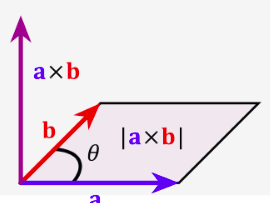==== # 2 矩阵 ## 2.1 定义 • 将一些元素排列成若干行，每行放上相同数量的元素，就是一个矩阵。这里说的元素可以是数字，例如以下的矩阵： A = [ 9 13 5 1 11 7 3 9 2 6 0 7 ] \mathbf{A} = \begin{bmatrix} 9 & 13 & 5 \\ 1 & 11 & 7 \\ 3 & 9 & 2 \\ 6 & 0 & 7 \end{bmatrix} 排列成的形状是矩形，所以称为矩阵。在中国大陆，横向的元素组称为“行”，纵向称为“列”， • 行数是1或列数是1的矩阵又可分别称为行向量和列向量。这是因为一个向量可以表示成行数或列数是1的矩阵形式。矩阵的任一行／列都是一个行／列向量 ## 2.2 矩阵的基本运算 • 矩阵的最基本运算包括矩阵加（减）法，数乘和转置运算。被称为“矩阵加法”、“数乘”和“转置”的运算不止一种，其中最基本最常用的定义如下：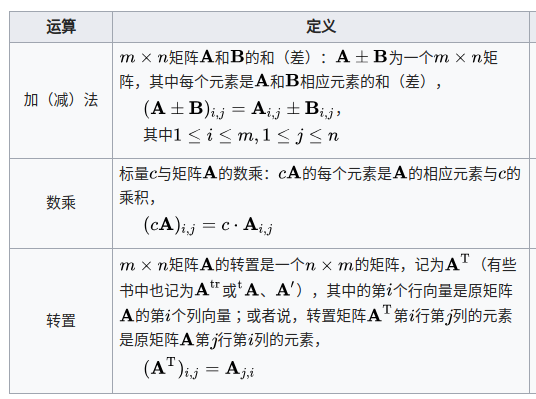• 矩阵的加法运算满足交换律：\mathbf {A} +\mathbf {B} =\mathbf {B} +\mathbf {A} }。矩阵的转置和数乘运算对加法满足分配律： ( A + B ) T = A T + B T (\mathbf {A} +\mathbf {B} )^{\mathrm {T} }=\mathbf {A} ^{\mathrm {T} }+\mathbf {B} ^{\mathrm {T} }} c ( A + B ) = c A + c B c(\mathbf {A} +\mathbf {B} )=c\mathbf {A} +c\mathbf {B} } • 矩阵加法和数乘两种运算使得 M ( m , n , R ) {\mathcal {M}}(m,n,\mathbb {R} )} 成为一个 m n mn} 维的实数线性空间。而转置和数乘运算满足类似于结合律的规律： c ( A T ) = c ( A ) T c(\mathbf {A} ^{\mathrm {T} })=c(\mathbf {A} )^{\mathrm {T} }} • 矩阵也有类似行列式的初等变换，即对矩阵的某些行和某些列进行三类操作：交换两行／列，将一行／列的每个元素都乘以一个固定的量，以及将一行／列的每个元素乘以一个固定的量之后加到另一行／列的相应元素上。这些操作在求其逆矩阵时有用。 ## 2.3 矩阵乘法 • 两个矩阵的乘法仅当第一个矩阵 A \mathbf {A} } 的列数(column)和另一个矩阵 B \mathbf {B} } 的行数(row)相等时才能定义。如 A \mathbf {A} } m × n m\times n} 矩阵和 B \mathbf {B} } n × p n\times p} 矩阵，它们的乘积 A B \mathbf {AB} } 是一个 m × p m\times p} 矩阵，它的一个元素: [ A B ] i , j = A i , 1 B 1 , j + A i , 2 B 2 , j + ⋯ + A i , n B n , j = ∑ r = 1 n A i , r B r , j { [\mathbf{AB}]_{i,j} = A_{i,1}B_{1,j} + A_{i,2}B_{2,j} + \cdots + A_{i,n}B_{n,j} = \sum_{r=1}^n A_{i,r}B_{r,j}} 例如: [ 1 0 2 − 1 3 1 ] × [ 3 1 2 1 1 0 ] = [ ( 1 × 3 + 0 × 2 + 2 × 1 ) ( 1 × 1 + 0 × 1 + 2 × 0 ) ( − 1 × 3 + 3 × 2 + 1 × 1 ) ( − 1 × 1 + 3 × 1 + 1 × 0 ) ] = [ 5 1 4 2 ] \begin{bmatrix} 1 & 0 & 2 \\ -1 & 3 & 1 \\ \end{bmatrix} \times \begin{bmatrix} 3 & 1 \\ 2 & 1 \\ 1 & 0 \end{bmatrix} = \begin{bmatrix} (1 \times 3 + 0 \times 2 + 2 \times 1) & (1 \times 1 + 0 \times 1 + 2 \times 0) \\ (-1 \times 3 + 3 \times 2 + 1 \times 1) & (-1 \times 1 + 3 \times 1 + 1 \times 0) \\ \end{bmatrix} = \begin{bmatrix} 5 & 1 \\ 4 & 2 \\ \end{bmatrix} • 矩阵的乘法满足结合律和对矩阵加法的分配律（左分配律和右分配律）： 结合律： ( A B ) C = A ( B C ) (\mathbf {AB} )\mathbf {C} =\mathbf {A} (\mathbf {BC} )} 左分配律： ( A + B ) C = A C + B C (\mathbf {A} +\mathbf {B} )\mathbf {C} =\mathbf {AC} +\mathbf {BC} } 右分配律： C ( A + B ) = = C A + C B C(A + B) =} = CA + CB . • 矩阵的乘法与数乘运算之间也满足类似结合律的规律；与转置之间则满足倒置的分配律。 c ( A B ) = ( c A ) B = A ( c B ) c(\mathbf {AB} )=(c\mathbf {A} )\mathbf {B} =\mathbf {A} (c\mathbf {B} )} ( A B ) T = B T A T (\mathbf {AB} )^{\mathrm {T} }=\mathbf {B} ^{\mathrm {T} }\mathbf {A} ^{\mathrm {T} }} • 矩阵乘法不满足交换律: A B ≠ B A \mathbf {AB} \neq \mathbf {BA} } ## 2.4 线性方程组 • 矩阵乘法的一个基本应用是在线性方程组上。线性方程组是方程组的一种，它符合以下的形式： { a 1 , 1 x 1 + a 1 , 2 x 2 + ⋯ + a 1 , n x n = b 1 a 2 , 1 x 1 + a 2 , 2 x 2 + ⋯ + a 2 , n x n = b 2 ⋮ ⋮ a m , 1 x 1 + a m , 2 x 2 + ⋯ + a m , n x n = b m \begin{cases}a_{1,1}x_{1} + a_{1,2}x_{2} + \cdots + a_{1,n}x_{n}= b_{1} \\ a_{2,1}x_{1} + a_{2,2}x_{2} + \cdots + a_{2,n}x_{n}= b_{2} \\ \vdots \quad \quad \quad \vdots \\ a_{m,1}x_{1} + a_{m,2}x_{2} + \cdots + a_{m,n}x_{n}= b_{m} \end{cases} • 其中的 a 1 , 1 , a 1 , 2 a_{1,1},\,a_{1,2}} 以及 b 1 , b 2 b_{1},\,b_{2}} 等等是已知的常数，而 x 1 , x 2 x_{1},\,x_{2}} 等等则是要求的未知数。运用矩阵的方式，可以将线性方程组写成一个向量方程： A x = b \mathbf{A} \mathbf{x} = \mathbf{b} 其中， A \mathbf {A} } 是由方程组里未知量的系数排成的 m × n m\times n} 矩阵， x \mathbf {x} } 是含有 n n} 个元素的行向量， b \mathbf {b} } 是含有 m m} 个元素的行向量: A = [ a 1 , 1 a 1 , 2 ⋯ a 1 , n a 2 , 1 a 2 , 2 ⋯ a 2 , n ⋮ ⋮ ⋱ ⋮ a m , 1 a m , 2 ⋯ a m , n ] , x = [ x 1 x 2 ⋮ x n ] , b = [ b 1 b 2 ⋮ b m ] \mathbf{A} = \begin{bmatrix} a_{1,1} & a_{1,2} & \cdots & a_{1,n} \\ a_{2,1} & a_{2,2} & \cdots & a_{2,n} \\ \vdots & \vdots & \ddots & \vdots \\ a_{m,1} & a_{m,2} & \cdots & a_{m,n} \end{bmatrix},\quad \mathbf{x} = \begin{bmatrix} x_1 \\ x_2 \\ \vdots \\ x_n \end{bmatrix},\quad \mathbf{b} = \begin{bmatrix} b_1 \\ b_2 \\ \vdots \\ b_m \end{bmatrix} ## 2.5 线性变换 • 矩阵是线性变换的便利表达法。矩阵乘法的本质在联系到线性变换的时候最能体现，因为矩阵乘法和线性变换的合成有以下的联系： 以 R n \mathbb {R} ^{n}} 表示所有长度为 n n} 的行向量的集合。每个 m × n m\times n} 的矩阵 A \mathbf {A} } 都代表了一个从 R n \mathbb {R} ^{n}} 射到 R m \mathbb {R} ^{m}} 的线性变换。 • 反过来，对每个线性变换 f : R n → R m f:\mathbb {R} ^{n}\rightarrow \mathbb {R} ^{m}} ，都存在唯一m×n矩阵 A f \mathbf {A} _{f}} 使得对所有 R n \mathbb {R} ^{n}} 中的元素 x x} f ( x ) = A f x f(x)=A_{f}x} 。这个矩阵 A f \mathbf {A} _{f}} i i} 行第 j j} 列上的元素是正则基向量 e j = ( 0 , ⋯ , 0 , 1 , 0 , ⋯ 0 ) T \mathbf {e} _{j}=(0,\cdots ,0,1,0,\cdots 0)^{T}} (第j个元素是1，其余元素是0的向量）在 f f} 映射后的向量 f ( e j ) f(\mathbf {e} _{j})} 的第 i i} 个元素。 • 以下是一些典型的2维实平面上的线性变换对平面向量（图形）造成的效果，以及它们对应的2维矩阵。其中每个线性变换将蓝色图形映射成绿色图形；平面的原点(0, 0)用黑点表示。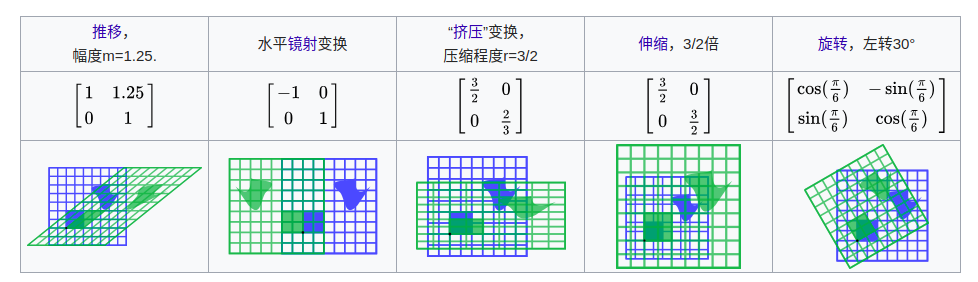• 矩阵的秩是指矩阵中线性无关的行／列向量的最大个数，同时也是矩阵对应的线性变换的像空间的维度。秩－零化度定理说明矩阵的列数量等于矩阵的秩与零空间维度之和。 ## 2.6 方块矩阵 ### 2.6.1 方阵 • 行数与列数相同的矩阵称为方块矩阵，简称方阵。所有 n n 维的方块矩阵构成一个线性空间，这个空间对矩阵乘法也是封闭的，因此也是一个代数。方阵 A \mathbf {A} } 称为可逆或非奇异的，如果存在另一个方阵 B \mathbf {B} } ，使得 A B = I n \mathbf {AB} =\mathbf {I} _{n}} 成立。这时候可以证明也有 B A = I n \mathbf {BA} =\mathbf {I} _{n}} 成立，可将矩阵 B \mathbf {B} } 称为 A \mathbf {A} } 的逆矩阵。一个矩阵 A \mathbf {A} } 的逆矩阵如果存在的话，就是唯一的，通常记作 A − 1 \mathbf {A} ^{-1}} • 矩阵 A \mathbf {A} } 的元素 A i , i A_{i,i}} 称为其主对角线上的元素。方块矩阵 A \mathbf {A} } 的所有主对角线元素之和称为它的迹，写作 t r ( A ) \mathrm {tr}}(\displaystyle A) 尽管矩阵的乘法不满足交换律，方阵相乘时交换顺序会导致乘积变化，但它们的迹不会变，即 t r ( A B ) = t r ( B A ) \mathrm {tr} (\mathbf {AB} )=\mathrm {tr} (\mathbf {BA} )} 矩阵转置的迹等于其自身的迹， t r ( A ) = t r ( A T ) \mathrm {tr} (\mathbf {A} )=\mathrm {tr} (\mathbf {A} ^{\mathrm {T} })} ### 2.6.2 行列式 • 方块矩阵 A \mathbf {A} } 的行列式是一个将其映射到标量的函数，记作 det ⁡ ( A ) \det(\mathbf {A} )} ∣ A ∣ \mathbf {|A|} } ，反映了矩阵自身的一定特性。一个方阵的行列式等于0当且仅当该方阵不可逆。系数是实数的时候，二维（三维）方阵 A \mathbf {A} } 的行列式的绝对值表示单位面积（体积）的图形经过 A \mathbf {A} } 对应的线性变换后得到的图形的面积（体积），而它的正负则代表了对应的线性变换是否改变空间的定向：行列式为正说明它保持空间定向，行列式为负则说明它逆转空间定向。 • 两个矩阵相乘，乘积的行列式等于它们的行列式的乘积： det ⁡ ( A B ) = det ⁡ ( A ) ⋅ det ⁡ ( B ) \det(\mathbf {AB} )=\det(\mathbf {A} )\cdot \det(\mathbf {B} )} 。将矩阵的一行／列乘以某个系数加到另一行／列上不改变矩阵的行列式，将矩阵的两行／列互换则使得其行列式变号。用这两种操作可以将矩阵变成一个上三角矩阵或下三角矩阵，而后两种矩阵的行列式就是主对角线上元素的乘积，因此能方便地计算。运用行列式可以计算线性方程组的解（见克莱姆法则）。 ### 2.6.3 特征值与特征向量 • n × n n\times n 的方块矩阵 A \mathbf {A} } 的一个特征值和对应特征向量是满足: A v = λ v {\mathbf {Av} =\lambda \mathbf {v} }} 的标量 λ \lambda } 以及非零向量 v \mathbf {v} } 。特征值和特征向量的概念对研究线性变换很有帮助。一个线性变换可以通过它对应的矩阵在向量上的作用来可视化。 • 一般来说，一个向量在经过映射之后可以变为任何可能的向量，而特征向量具有更好的性质。 • 假设在给定的基底下，一个线性变换对应着某个矩阵 A \mathbf {A} } ，如果一个向量 x \mathbf {x} } 可以写成矩阵的几个特征向量的线性组合： x = c 1 x λ 1 + c 2 x λ 2 + ⋯ + c k x λ k \mathbf {x} =c_{1}\mathbf {x} _{\lambda _{1}}+c_{2}\mathbf {x} _{\lambda _{2}}+\cdots +c_{k}\mathbf {x} _{\lambda _{k}}} • 其中的 x λ i \mathbf {x} _{\lambda _{i}}} 表示此向量对应的特征值是 λ i \lambda _{i}} ，那么向量 x \mathbf {x} } 经过线性变换后会变成： A x = c 1 λ 1 x λ 1 + c 2 λ 2 x λ 2 + ⋯ + c k λ k x λ k \mathbf{Ax} = c_1 \lambda_1 \mathbf{x}_{\lambda_1} + c_2 \lambda_2 \mathbf{x}_{\lambda_2} + \cdots + c_k \lambda_k \mathbf{x}_{\lambda_k} 可以清楚地知道变换后向量的结构。 ### 2.6.4 对称 • 对称 ： A = A T \mathbf {A} =\mathbf {A} ^{\mathrm {T} }} • 反对称 ： A = − A T \mathbf {A} =- \mathbf {A} ^{\mathrm {T} }} • 在复系数矩阵中，则有埃尔米特矩阵的概念：满足 A = A ∗ \mathbf {A} =\mathbf {A} ^{*}} 的方块矩阵称为埃尔米特矩阵，其中的 A ∗ \mathbf {A} ^{*}} 表示 A \mathbf {A} } 的共轭转置矩阵。 ### 2.6.5 正定性 n × n n\times n 的实对称矩阵 A \mathbf {A} } 如果满足对所有非零向量 x ∈ R n \mathbf {x} \in \mathbf {R} ^{n}} ，对应的二次型 Q ( x ) = x T A x Q(\mathbf {x} )=\mathbf {x} ^{\mathrm {T} }\mathbf {Ax} } 函数值都是正数，就称 \mathbf {A} } \mathbf{A}为正定矩阵。类似地还有半正定矩阵、负定矩阵、不定矩阵等概念。对称矩阵的正定性与其特征值密切相关。矩阵是正定的当且仅当其特征值都是正数。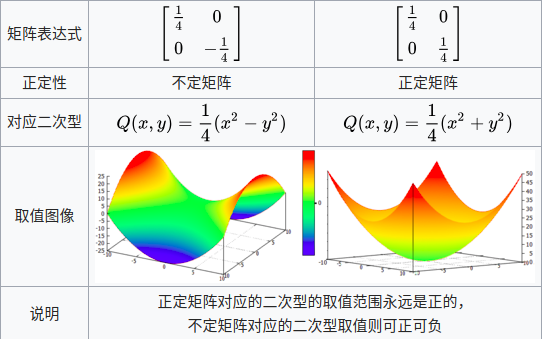## 2.7 矩阵的计算 A − 1 = Adj ⁡ ( A ) det ⁡ ( A ) \mathbf {A} ^{-1}={\frac {\operatorname {Adj} (\mathbf {A} )}{\det(\mathbf {A} )}}} ### 2.7.1 矩阵分解 • 矩阵研究的一大方向是将一般的矩阵用一些比较“简单”的矩阵来表示。这种表示方式称为矩阵的变换与分解。矩阵变换与分解的方法有很多，它们的目的都是希望化简后的矩阵保持原矩阵的某些性质，比如行列式、秩或逆矩阵，而形式相对简单，因而能用容易地进行讨论和计算，或者能使得某些算法更易执行。 • LU分解将矩阵分解为一个下三角矩阵 \mathbf {L} } \mathbf {L} 和一个上三角矩阵 \mathbf {U} } \mathbf{U}的乘积。 例如解线性方程组时，如果将系数矩阵 A \mathbf {A} } 分解成 A = L U \mathbf {A} =\mathbf {LU} } 的形式，那么方程的求解可以分解为求解 L y = b \mathbf {Ly} =\mathbf {b} } U x = y \mathbf {Ux} =\mathbf {y} } 两步，而后两个方程可以十分简洁地求解 • 高斯消元法也是一种矩阵分解方法。通过初等变换操作，可以将任何矩阵变为阶梯形矩阵，而每个操作可以看做是将矩阵乘上一个特定的初等矩阵 奇异值分解则是另一种分解方法，将一个矩阵表示成3个矩阵的乘积： A = U D V \mathbf {A} =\mathbf {UDV} } 。其中 U \mathbf {U} } V \mathbf {V} } 是酉矩阵， \mathbf {D} } \mathbf {D} 是对角矩阵。 A n = ( P D P − 1 ) n = P D P − 1 P D P − 1 … P D P − 1 = P D n P − 1 \mathbf {A} ^{n}=(\mathbf {PDP} ^{-1})^{n}=\mathbf {PDP} ^{-1}\mathbf {PDP} ^{-1}\ldots \mathbf {PDP} ^{-1}=\mathbf {PD} ^{n}\mathbf {P} ^{-1}} ## 2.8 矩阵的推广 • 无限维矩阵 • 空矩阵 • 分块矩阵 ## 2.9 雅克比矩阵 雅克比矩阵 J = [ ∂ f ∂ x 1 ⋯ ∂ f ∂ x n ] = [ ∂ f 1 ∂ x 1 ⋯ ∂ f 1 ∂ x n ⋮ ⋱ ⋮ ∂ f m ∂ x 1 ⋯ ∂ f m ∂ x n ] \mathbf {J} ={\begin{bmatrix}{\dfrac {\partial \mathbf {f} }{\partial x_{1}}}&\cdots &{\dfrac {\partial \mathbf {f} }{\partial x_{n}}}\end{bmatrix}}={\begin{bmatrix}{\dfrac {\partial f_{1}}{\partial x_{1}}}&\cdots &{\dfrac {\partial f_{1}}{\partial x_{n}}}\\\vdots &\ddots &\vdots \\{\dfrac {\partial f_{m}}{\partial x_{1}}}&\cdots &{\dfrac {\partial f_{m}}{\partial x_{n}}}\end{bmatrix}}} # 3.共轭转置 • 矩阵 A A} 的共轭转置 A ∗ A^{*}} 又称埃尔米特共轭、埃尔米特转置，英语：conjugate transpose）定义为： ( A ∗ ) i , j = A j , i ‾ {(A^*)_{i,j} = \overline{A_{j,i}}} • 其中 ( ⋅ ) i , j (\cdot )_{i,j}} 表示矩阵i行j列上的元素， ( ⋅ ) ‾ {\overline {(\cdot )}}} 表示标量的复共轭。 A ∗ = ( A ‾ ) T = A T ‾ A^* = (\overline{A})^\mathrm{T} = \overline{A^\mathrm{T}} • 其中 A T ⁣ A^{\mathrm {T} }\,\!} 是矩阵A的转置， A ‾ ⁣ {\overline {A}}\,\!} 表示对矩阵A中的元素取复共轭。 • 通常用以下记号表示矩阵A的共轭转置： A ∗ ⁣ A^* \,\! A H ⁣ A^{\mathrm {H} }\,\!} 常用于线性代数 A † ⁣ A^{\dagger }\,\!} 普遍用于量子力学 A + ⁣ A^{+}\,\!} （但这一记号通常指矩阵的摩尔－彭若斯广义逆) • 注意：某些情况下 A ∗ ⁣ A^{*}\,\!} 也指仅对矩阵元素取复共轭，而不做矩阵转置，切勿混淆。 ## 3.1 实数 如果A的元素是实数，那么 A ∗ A^* 与A的转置 A T A^T 相等。把复值方块矩阵视为复数的推广，以及把共轭转置视为共轭复数的推广通常是非常有用的。 展开全文• 矩阵基础 |共轭转置Conjugate transpose 操作方法性质 ) 操作方法 性质 一、操作方法 A中的每个元素aija_{ij}aij​取共轭得bijb_{ij}bij​，将新得到的由bijb_{ij}bij​组成的新m*n型矩阵记为矩阵B，再对矩阵B作... • 证明：\(Rank(A) = Rank(A^HA线性方程组 $$A^HAx = A^Hb$$ 恒有解其中 $$A^H$$ 为 $$A$$ 的共轭转置矩阵证明证明 $$Ax= 0$$ 和 $$A^HA x=0$$ 同解即可。因为对于 $$Ax=0, A\in R^(s\times n)$$，如果矩阵...
• 首先，我们回顾了低秩矩阵分解的概率模型中常用的一些概率分布，并介绍了一些概率分布的共轭先验以简化贝叶斯推断。 然后，我们为概率低秩矩阵分解提供了两种主要的推理方法，即吉布斯采样和变分贝叶斯推理。 接...
• 推论 设A是m×n矩阵，其满分解为 A=BCA=BCA=BC其中B是m×r矩阵，C是r×n矩阵，r(A)=r(B)=r©=r，则 A+=CH(CCH)−1(BHB)−1BH A^+=C^H\left( CC^H \right) ^{-1}\left( B^HB \right) ^{-1}B^H A+=CH(CCH)−1(BHB)...经验分享
• 前言：花了一个半月时间学习了 北大丘维声的《高等代数》、北理史荣昌的《矩阵分析》、清华张贤达的《矩阵分析与应用》；北大与哈工大的网课。本质：（万物皆矩阵矩阵论主要研究矩阵，对于图像、神经网络等可表示...
• 矩阵的谱分解(可对角化矩阵——满可逆)谱分解定理：设 为一个n阶可对角化矩阵，A的谱为 其中 的重数为 ,则存在唯一一组s个n阶方阵 ,满足(1) (2) (3) (4) (5) 这些矩阵 称为矩阵A的成分矩阵或主幂等矩阵。...
• 展开全部这是因为每个矩阵都可以通过初等变换，得到唯一的标准62616964757a686964616fe4b893e5b19e31333431343039型与之对应，而标准型中的非零行数就是。不管通过初等行变换来求行，还是初等列变换求列，最终...
• 展开全部对于任意矩阵A(甚至62616964757a686964616fe4b893e5b19e31333431346438是非方的)，A(T)A(这个时候就变成方阵了，可以算特征值了)的特征值就称为A的奇异值。奇异值有个特性，就是A(T)A和AA(T)特征值相同。...
• 我对numpy不是很有经验，但根据@hpaulj的评论，我可以提出以下建议：如果您不想受到numpy.matrix对象的限制(请参见警告here)，您可以定义自己的函数来执行共轭转置。你需要做的就是转置数组，然后从结果中减去结果的...
• MATLAB常见矩阵运算函数 1.转置 如矩阵A 转置后 2.求行列式的值det(A) 使用此函数必须保证A为方阵 3.求矩阵 4.求方阵的特征值 5.求方阵的逆矩阵matlab
• 该方法基于最小互信息准则,通过对行满分离矩阵的奇异值分解而引入了超定盲源分离的代价函数。利用共轭梯度优化算法推导出了迭代计算分离矩阵的更新公式。在每次迭代计算中,利用随机变量概率密度估计的核函数法在线...
• 初等变换与矩阵、向量组的矩阵的判定方法 向量空间、正交矩阵与线性变换 方阵的迹及其性质 矩阵的特征值 相似变换与相似对角化 复数的运算法则、复矩阵共轭共轭转置 复数的运算法则 ...机器学习的数学基础
• eye 单位矩阵 zeros 全零矩阵 ones 全1矩阵 rand 均匀分布随机阵 genmarkov 生成随机Markov矩阵 linspace 线性等分向量 logspace 对数等分向量 logm 矩阵对数运算 cumprod 矩阵元素累计乘 cumsum 矩阵元素累计和 ...
• ## 矩阵类型

千次阅读 2020-12-21 17:06:14
1. 幺正矩阵（酉矩阵） ...即幺正矩阵的逆矩阵就是其共轭转置矩阵U−1=U∗U^{-1}=U^*U−1=U∗幺正矩阵是实数上的正交矩阵在复数上的推广。 共轭转置：(A∗)ij=Aji‾(A^*)_{ij}=\overline{A_{ji}}(A∗)ij​=Amatrix
• 对角矩阵：主对角线之外的元素皆为0的矩阵。 数量矩阵：主对角线上的元素相等的对角矩阵。 单位矩阵：主对角线上的元素都为1的对角矩阵。 1. 提取矩阵的对角线元素(得到一个一维矩阵) diag(A)：提取矩阵A主对角线...matlab
• 依然延续我们在Hermite二次型这个系列的第(1)篇文章中提到的那样，矩阵论中Hermite二次型的相关讨论大多可以直接借鉴在线性代数中的思路。 因此，要对H二次型进行讨论，也会将其转变成H阵的相关问题。 一. 相关...数学
• ## Matlab 矩阵运算

千次阅读 2021-04-22 03:29:59
• 给出矩阵广义逆的定义(MP方程)以及相关定理，利用广义逆解决最小二乘问题。数学 最小二乘
• 转置：A.'为矩阵A的转置，A’为矩阵A的共轭转置； rot90(A,k)：将矩阵A逆时针方向旋转90°的k倍，k为1时可省略； fliplr(A)：将矩阵A左右翻转； flipld(A)：将矩阵A上下翻转。 矩阵的行列式、、迹 det(A)：求矩阵A...matlab
• 在数学中，正规矩阵 (英语: normal matrix) A\mathbf{A}A 是与自己的共轭转置满 足交换律的实系数方块矩阵，也就是说， A\mathbf{A}A 满足 A∗A=AA∗ \mathbf{A}^{*} \mathbf{A}=\mathbf{A} \mathbf{A}^{*} A∗A=AA...算法
• 矩阵基本知识一、矩阵的创建直接输入法利用Matlab函数创建矩阵利用文件创建矩阵二、矩阵的拆分矩阵元素矩阵拆分特殊矩阵三、矩阵的...矩阵与迹向量和矩阵的范数矩阵的特征值与特征向量五、字符串六、其他第二部分...
• 名称定义对角阵只有对角线上有非零元素的矩阵数量矩阵对角线上的元素全相等的对角阵单位矩阵对角线上的元素都为1的对角矩阵上三角形矩阵对角线以下的元素全为零的矩阵下三角形矩阵对角线以上的元素全为零的矩阵零......